matplotlib.pyplot.contourf¶

matplotlib.pyplot.contourf(*args, data=None, **kwargs)[source]

Plot contours.

Call signature:

contour([X, Y,] Z, [levels], **kwargs)

contour and contourf draw contour lines and filled contours, respectively. Except as noted, function signatures and return values are the same for both versions.

Notes

1. contourf differs from the MATLAB version in that it does not draw the polygon edges. To draw edges, add line contours with calls to contour.

2. contourf fills intervals that are closed at the top; that is, for boundaries z1 and z2, the filled region is:

z1 < Z <= z2

except for the lowest interval, which is closed on both sides (i.e. it includes the lowest value).

Examples using matplotlib.pyplot.contourf¶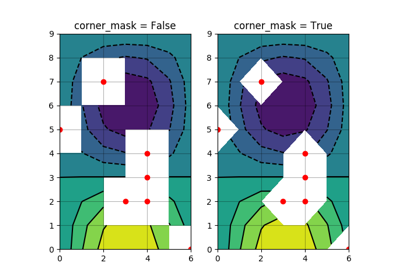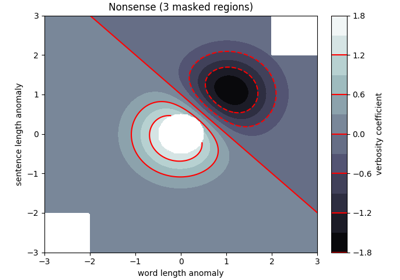Contourf Demo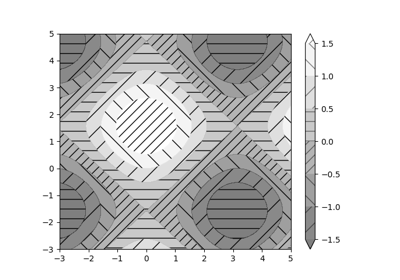Contourf Hatching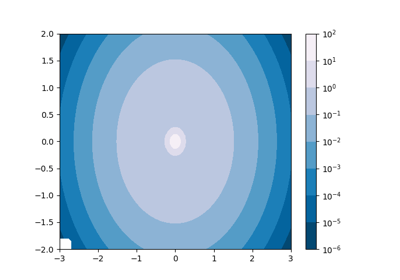Contourf and log color scale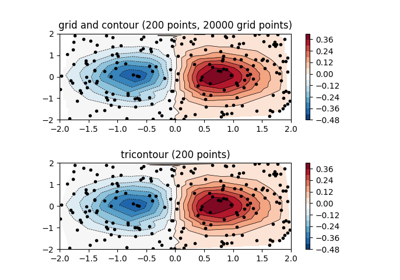Contour plot of irregularly spaced data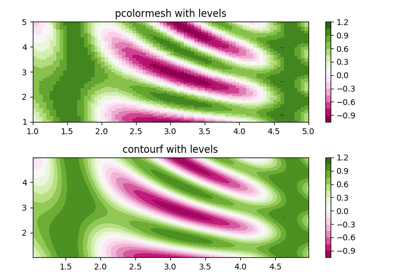pcolormesh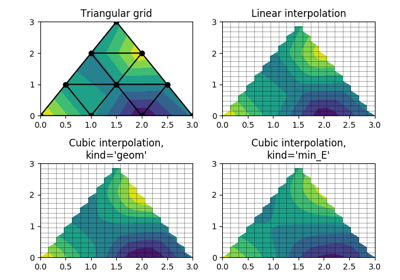Triinterp Demo